# How to use the ISBLANK function in Excel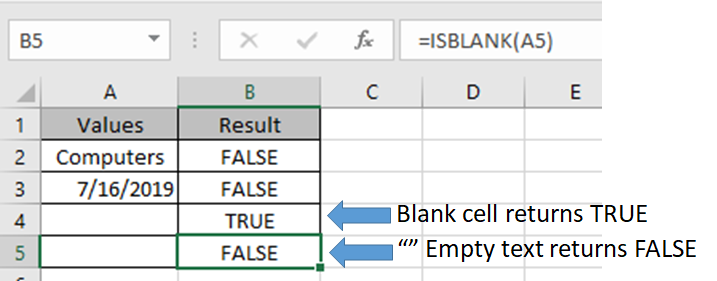In this article, we will learn How to use the ISBLANK function in Excel.

What is the ISBLANK function ?

The ISBLANK function is a logical operator to check if the cell is empty or not. If a cell has a formula, text, number percentage, date or currency then the ISBLANK function returns FALSE indicating that the cell is not blank. If and only if the cell has no value then only formula returns FALSE indicating that the cell has no value at all.

ISBLANK Function in Excel

ISBLANK function returns TRUE or FALSE having cell reference as input.

Syntax:

 =ISBLANK (cell_ref)

Cell_ref : cell reference of cell to check e.g. B3

Example :

All of these might be confusing to understand. Let's understand how to use the function using an example. Here We have some Values to test the function, except in the A5 cell. A5 cell has a formula that returns empty text.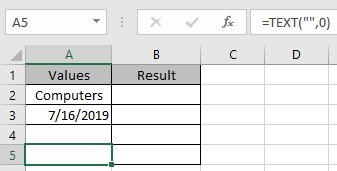Use the Formula

 =ISBLANK(A2)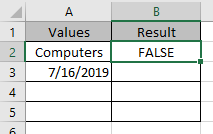It returns False as there is text in the A2 cell.

Applying the formula in other cells using Ctrl + D shortcut key.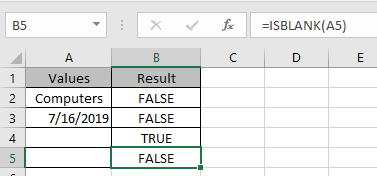You must be wondering why didn’t the function returns False. As A5 cell has a formula making it non blank.

USE of ISBLANK function along with other function like IF function

The use of ISBLANK function is generally used with other functions to check the cell and return corresponding results.

Let’s understand with an example here. Here we have some values on list 1 and we only want the formula to return the same value if not blank and empty value if blank.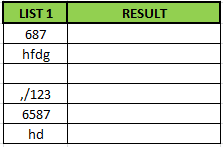Formula:

 =IF(ISBLANK(D5)=TRUE,"", D5)

Explanation:

ISBLANK : function checks the cell D5.

"" :  returns an empty string blank cell instead of Zero.

IF function performs a logic_test if the test is true, it returns an empty string else returns the same value.

The resulting output will be like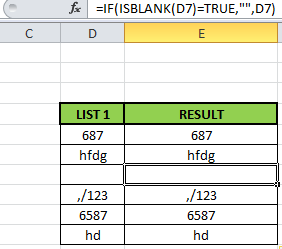As you can see the formula returns the same value or blank cell based on the logic_test.

Hope this article about How to use the ISBLANK function in Excel is explanatory. Find more articles on calculating values and related Excel formulas here. If you liked our blogs, share it with your friends on Facebook. And also you can follow us on Twitter and Facebook. We would love to hear from you, do let us know how we can improve, complement or innovate our work and make it better for you. Write to us at info@exceltip.com.

Related Articles :

Adjusting a Formula to Return a Blank : Formula adjust its result on the basis of whether the cell is blank or not. Click the link to know how to use ISBLANK function with IF function in Excel.

Checking Whether Cells in a Range are Blank, and Counting the Blank Cells : If cells in a range are blank and calculating the blank cells in Excel then follow the link to understand more.

How to Calculate Only If Cell is Not Blank in Excel : Calculate only and only if the cell is not blank then use ISBLANK function in Excel.

Delete drop down list in Excel : The dropdown list is used to restrict the user to input data and gives the option to select from the list. We need to delete or remove the Dropdown list as the user will be able to input any data instead of choosing from a list.

Deleting All Cell Comments in Excel 2016 : Comments in Excel to remind ourselves and inform someone else about what the cell contains. To add the comment in a cell, Excel 2016 provides the insert Comment function. After inserting the comment it is displayed with little red triangles.

How to Delete only Filtered Rows without the Hidden Rows in Excel :Many of you are asking how to delete the selected rows without disturbing the other rows. We will use the Find & Select option in Excel.

How to Delete Sheets Without Confirmation Prompts Using VBA In Excel :There are times when we have to create or add sheets and later on we find no use of that sheet, hence we get the need to delete sheets quickly from the workbook

Popular Articles :

How to use the IF Function in Excel : The IF statement in Excel checks the condition and returns a specific value if the condition is TRUE or returns another specific value if FALSE.

How to use the VLOOKUP Function in Excel : This is one of the most used and popular functions of excel that is used to lookup value from different ranges and sheets.

How to use the SUMIF Function in Excel : This is another dashboard essential function. This helps you sum up values on specific conditions.

How to use the COUNTIF Function in Excel : Count values with conditions using this amazing function. You don't need to filter your data to count specific values. Countif function is essential to prepare your dashboard.

Terms and Conditions of use

The applications/code on this site are distributed as is and without warranties or liability. In no event shall the owner of the copyrights, or the authors of the applications/code be liable for any loss of profit, any problems or any damage resulting from the use or evaluation of the applications/code.